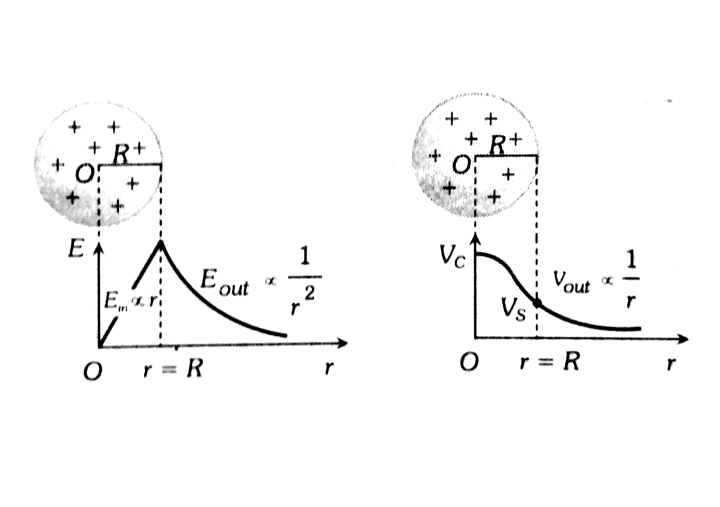Q

# Need explanation for: In a uniformly charged sphere of total charge Q and radius R the electric field E is plotted as a function of distance from the centre. The graph which would correspond to the above will be :

In a uniformly charged sphere of total charge Q and radius R the electric field E is plotted as a function of distance from the centre. The graph which would correspond to the above will be :

• Option 1)• Option 2)• Option 3)• Option 4)93 Views
N

:    For uniformly charged sphere

As we discussed in

Graph - - whereinThe variation of  E   with distance  r   from the centre is as shown.Option 1)Option 2)Option 3)Option 4)Exams
Articles
Questions